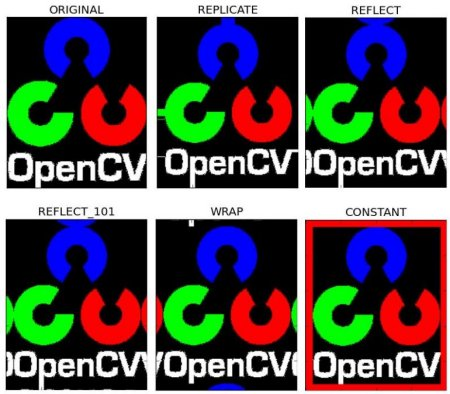OpenCV  4.1.0 Open Source Computer Vision
Basic Operations on Images

## Goal

Learn to:

• Access pixel values and modify them
• Access image properties
• Setting Region of Interest (ROI)
• Splitting and Merging images

Almost all the operations in this section is mainly related to Numpy rather than OpenCV. A good knowledge of Numpy is required to write better optimized code with OpenCV.

*( Examples will be shown in Python terminal since most of them are just single line codes )*

## Accessing and Modifying pixel values

Let's load a color image first:

>>> import numpy as np
>>> import cv2 as cv

You can access a pixel value by its row and column coordinates. For BGR image, it returns an array of Blue, Green, Red values. For grayscale image, just corresponding intensity is returned.

>>> px = img[100,100]
>>> print( px )
[157 166 200]
# accessing only blue pixel
>>> blue = img[100,100,0]
>>> print( blue )
157

You can modify the pixel values the same way.

>>> img[100,100] = [255,255,255]
>>> print( img[100,100] )
[255 255 255]

warning

Numpy is a optimized library for fast array calculations. So simply accessing each and every pixel values and modifying it will be very slow and it is discouraged.

Note
The above method is normally used for selecting a region of an array, say the first 5 rows and last 3 columns. For individual pixel access, the Numpy array methods, array.item() and array.itemset() are considered better, however they always return a scalar. If you want to access all B,G,R values, you need to call array.item() separately for all.

Better pixel accessing and editing method :

# accessing RED value
>>> img.item(10,10,2)
59
# modifying RED value
>>> img.itemset((10,10,2),100)
>>> img.item(10,10,2)
100

## Accessing Image Properties

Image properties include number of rows, columns and channels, type of image data, number of pixels etc.

The shape of an image is accessed by img.shape. It returns a tuple of number of rows, columns, and channels (if image is color):

>>> print( img.shape )
(342, 548, 3)
Note
If an image is grayscale, the tuple returned contains only the number of rows and columns, so it is a good method to check whether the loaded image is grayscale or color.

Total number of pixels is accessed by img.size:

>>> print( img.size )
562248

Image datatype is obtained by img.dtype:

>>> print( img.dtype )
uint8
Note
img.dtype is very important while debugging because a large number of errors in OpenCV-Python code is caused by invalid datatype.

## Image ROI

Sometimes, you will have to play with certain region of images. For eye detection in images, first face detection is done all over the image. When a face is obtained, we select the face region alone and search for eyes inside it instead of searching the whole image. It improves accuracy (because eyes are always on faces :D ) and performance (because we search in a small area).

ROI is again obtained using Numpy indexing. Here I am selecting the ball and copying it to another region in the image:

>>> ball = img[280:340, 330:390]
>>> img[273:333, 100:160] = ball

Check the results below:image

## Splitting and Merging Image Channels

Sometimes you will need to work separately on B,G,R channels of image. In this case, you need to split the BGR images to single channels. In other cases, you may need to join these individual channels to a BGR image. You can do it simply by:

>>> b,g,r = cv.split(img)
>>> img = cv.merge((b,g,r))

Or

>>> b = img[:,:,0]

Suppose you want to set all the red pixels to zero, you do not need to split the channels first. Numpy indexing is faster:

>>> img[:,:,2] = 0

Warning

cv.split() is a costly operation (in terms of time). So do it only if you need it. Otherwise go for Numpy indexing.

## Making Borders for Images (Padding)

If you want to create a border around the image, something like a photo frame, you can use cv.copyMakeBorder(). But it has more applications for convolution operation, zero padding etc. This function takes following arguments:

• src - input image
• top, bottom, left, right - border width in number of pixels in corresponding directions
• borderType - Flag defining what kind of border to be added. It can be following types:
• value - Color of border if border type is cv.BORDER_CONSTANT

Below is a sample code demonstrating all these border types for better understanding:

import cv2 as cv
import numpy as np
from matplotlib import pyplot as plt
BLUE = [255,0,0]
replicate = cv.copyMakeBorder(img1,10,10,10,10,cv.BORDER_REPLICATE)
reflect = cv.copyMakeBorder(img1,10,10,10,10,cv.BORDER_REFLECT)
reflect101 = cv.copyMakeBorder(img1,10,10,10,10,cv.BORDER_REFLECT_101)
wrap = cv.copyMakeBorder(img1,10,10,10,10,cv.BORDER_WRAP)
constant= cv.copyMakeBorder(img1,10,10,10,10,cv.BORDER_CONSTANT,value=BLUE)
plt.subplot(231),plt.imshow(img1,'gray'),plt.title('ORIGINAL')
plt.subplot(232),plt.imshow(replicate,'gray'),plt.title('REPLICATE')
plt.subplot(233),plt.imshow(reflect,'gray'),plt.title('REFLECT')
plt.subplot(234),plt.imshow(reflect101,'gray'),plt.title('REFLECT_101')
plt.subplot(235),plt.imshow(wrap,'gray'),plt.title('WRAP')
plt.subplot(236),plt.imshow(constant,'gray'),plt.title('CONSTANT')
plt.show()

See the result below. (Image is displayed with matplotlib. So RED and BLUE channels will be interchanged):image